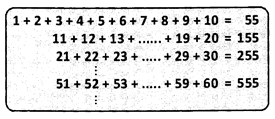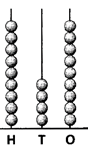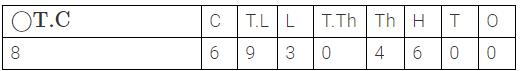Courses

# Olympiad Test: Knowing Our Numbers

## 20 Questions MCQ Test Mathematics (Maths) Class 6 | Olympiad Test: Knowing Our Numbers

Description
This mock test of Olympiad Test: Knowing Our Numbers for Class 6 helps you for every Class 6 entrance exam. This contains 20 Multiple Choice Questions for Class 6 Olympiad Test: Knowing Our Numbers (mcq) to study with solutions a complete question bank. The solved questions answers in this Olympiad Test: Knowing Our Numbers quiz give you a good mix of easy questions and tough questions. Class 6 students definitely take this Olympiad Test: Knowing Our Numbers exercise for a better result in the exam. You can find other Olympiad Test: Knowing Our Numbers extra questions, long questions & short questions for Class 6 on EduRev as well by searching above.
QUESTION: 1

### Observe the following pattern for obtaining the sums, and find the sum of numbers from 781 to 790.Solution:

Observing the pattern, we see that the last two digits are 55 and the left-most digits is/are the two left-most digits of the number in the given sums. Thus, 781 + 782 + .... + 789 + 790 = 7855.

QUESTION: 2

### In numbers from 1 to 100, how many times does the digit 0 appear?

Solution:

Numbers having 0 in numbers from 1 to 100 are 10, 20, 30, 40, 50, 60, 70, 80, 90 and 100. So, 0 appears 11 times.

QUESTION: 3

### How many numbers are there containing 2 digits?

Solution:

Number of two digit numbers = (The greatest two digit number - The smallest two digit number) + 1 =(99-10)+1 =90

QUESTION: 4

How many beads should be removed from the hundreds place in the abacus shown if it has to represent a number between 550 and 650?Solution:

The number represented by the abacus given is 949. To represent a number between 550 and 650, (i.e., 649) we need to remove 3 beads from the hundreds place.

QUESTION: 5

Which of the following is the correct expansion of 27012?

Solution:

To get the expanded form of a given number, multiply each of its digits with their respective place values and write the products as a sum. 27012 = 20000 + 7000 +0+10+2

QUESTION: 6

Which of the following is the greatest?

Solution:

The order from biggest to smallest number is  7221, 7212 , 7211 and the smallest is 7122.

QUESTION: 7

Choose the correct ascending order of 6411, 6144, 6344, 6744.

Solution:

Ascending order is
6144, 6344, 6411, 6744

QUESTION: 8

The smallest 5-digit number formed by using the digits 5,0,3,1 and 2 only once

Solution:

Arrange the numbers in incresing order which is
0,1,2,3,5 but 01235 is a 4 digit number and not 5 digit number so

QUESTION: 9

Write the greatest 4-digit number using different digits with 6 in the tens place.

Solution:

As we have to keep 6 at tens place we will freeze it at tens place
Th H T O

9 8 (6) 7

QUESTION: 10

Round 3500 to the nearest thousand.

Solution:

3500, 3600, 3700, 3800 and 3900 round up to 4000. To round a number to the nearest thousand, the digit in hundreds place is considered. If > = 5, rounded up and if < 5 rounded down. To round a number to the nearest ten thousand, the digit in thousands place is considered.

QUESTION: 11

Which of the following is the largest 8-digit number?

Solution:

Take '9' in all 8-digits to write the largest 8-digit number.

QUESTION: 12

Which is the smallest 9-digit number?

Solution:

To write the smallest n-digit number write 1 followed by (n - 1) zeros.

QUESTION: 13

What is the place value of 8 in the number 86,93,04,600?

Solution:8×10,00,00,000=80,00,00,000=Eighty crores

QUESTION: 14

How many thousands is 1 lakh?

Solution:

100 thousand makes up to 1 Lakh.

QUESTION: 15

Which is the place that comes on the immediate right of the hundreds place in the place value chart?

Solution:
QUESTION: 16

What are the places in the millions period?

Solution:

Million Period consists of places : Million, Hundred Millions, Thousand Millions

→M,HM,TM

QUESTION: 17

Under which period is ten lakhs?

Solution:

Ten lakhs & lakhs places are in the lakhs period.

QUESTION: 18

How many millions are there in 10 crores?

Solution:

10 crores (or 1000 lakhs) is equal to 100 million

As 1 million= 10 lakh and 1000 lakh/10 lakh = 100

QUESTION: 19

Identify the symbol to be placed in the box 100002 □ 1000002.

Solution:

100002= One lakh two

1000002= ten lakh two, So 100002 is smaller than 1000002

And hence 100002 < 1000002.

QUESTION: 20

How many crores make 1 billion?

Solution:

one billioion is 1,000,000,000 which is qual to 100 crores.